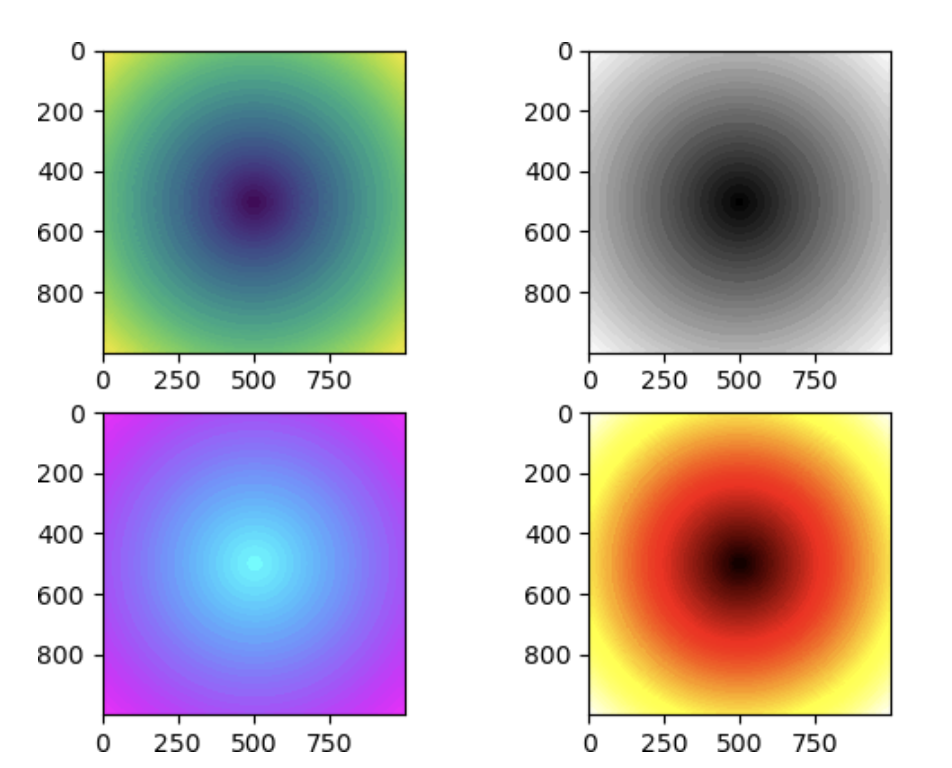# Matplotlib image图像处理

Matplotlib 软件包中的image模块提供了加载、缩放和显示图像的功能，该模块只能支持 PNG 格式的图片，如果格式不符，需要对图片的格式进行转换。

Matplotlib 支持的图片格式非常有限，所以通常情况下，建议采用 Python 图像处理库 Pillow 来处理图像，若感兴趣可以自行了解。

下面示例，imread() 函数用于读取图像数据并形成 ndarray 数组 ，其数据类型为 float32.

``````import matplotlib.pyplot as plt
import matplotlib.image as mpimg
import numpy as np

假设在当前目录中存在名为 mtplogo.png 的图像。通过执行 imsave() 函数，可以将包含图像数据的 ndarray 数组保存到磁盘文件中。

``plt.imsave("logo.png", img, cmap = 'gray', origin = 'lower')``

如下所示，将 imsave()方法的origin参数设置为 lower 实现了原始图像的垂直翻转。通过 imshow() 函数可以在 Matplotlib 查看器上绘制出相应的图像。其函数格式如下：

``imgplot = plt.imshow(img)``

下面列举一组示例：

``````import matplotlib.pyplot as plt
import numpy as np
fig=plt.figure()
#准备数据
#绘制z = sqrt(x^2+y^2)图像
points=np.arange(-5,5,0.01)
# meshgrid 接受两个一维数组，然后产生两个二维矩阵
xs,ys=np.meshgrid(points,points)
#绘制图像
z=np.sqrt(xs**2+ys**2)
#默认
ax.imshow(z)
ax.imshow(z,cmap = "gray")
ax.imshow(z,cmap="cool")
ax.imshow(z,cmap="hot")
#显示图像
plt.show()``````

输出结果如下：以上就是小编为大家整理发布的“Matplotlib image图像处理”一文，更多相关内容尽在开课吧广场Python教程频道。有用分享